Courses

# Digital Electronics - MCQ Test - 2

## 20 Questions MCQ Test GATE ECE (Electronics) 2022 Mock Test Series | Digital Electronics - MCQ Test - 2

Description
This mock test of Digital Electronics - MCQ Test - 2 for GATE helps you for every GATE entrance exam. This contains 20 Multiple Choice Questions for GATE Digital Electronics - MCQ Test - 2 (mcq) to study with solutions a complete question bank. The solved questions answers in this Digital Electronics - MCQ Test - 2 quiz give you a good mix of easy questions and tough questions. GATE students definitely take this Digital Electronics - MCQ Test - 2 exercise for a better result in the exam. You can find other Digital Electronics - MCQ Test - 2 extra questions, long questions & short questions for GATE on EduRev as well by searching above.
*Answer can only contain numeric values
QUESTION: 1

### In the circuit sho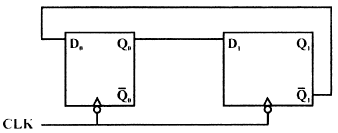Qo = Q1 = 0 Then the values of QO and QI after 335th clock pulse are _____________

Solution:

It is a MOD - 4 counter, because Johnson counter =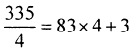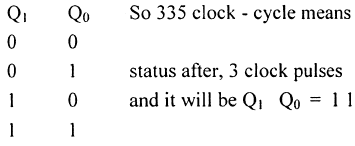QUESTION: 2

### An R — 2R ladder type DAC is shown in Figure if a switch status is '0', then 0 volt is applied and If a switch status is '1' then 5 volt is applied to the corresponding terminal of DAC.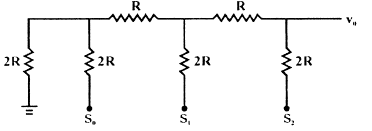What is value of output voltage V0 for switch status so = 0, s1= 1, s2 = 1.

Solution: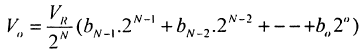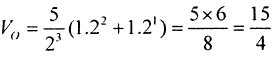QUESTION: 3

### An R — 2R ladder type DAC is shown in Figure if a switch status is '0', then 0 volt is applied and If a switch status is '1' then 5 volt is applied to the corresponding terminal of DAC.Q. What is the step size of DAC.

Solution:

Step size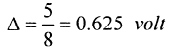QUESTION: 4

For the given circuit shown in figure signal generated at the output of AND gat is Y. there clock has signal frequency of 4 kHz, with duty cycle 50%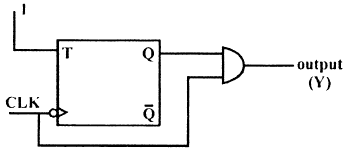Q. What is the value of frequency of output Y

Solution:

A FF is simply MOD-2 counter.

QUESTION: 5

The following waveform pattern is for a(n) ________.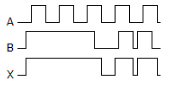Solution:
QUESTION: 6

The input X for count sequence 0, 2, 3, 1, 0, 2 ....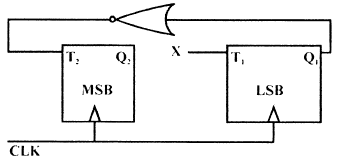Solution:

Just Solve by K-Map.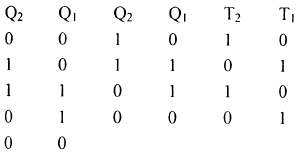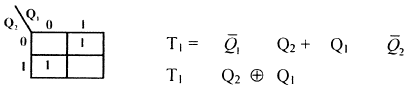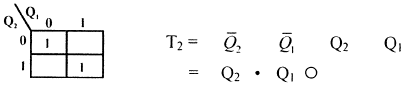QUESTION: 7

For a Flip-flop formed from 2 NAND gates as shown in figure, the unusable state corresponds to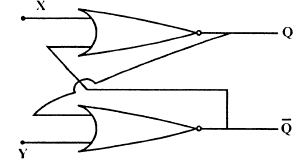Solution:

when both X = Y = 0 then value of Q &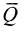will remain same so unstable.

QUESTION: 8

In the figure as long as XI = 1 and X2 = 1 the output Q remains at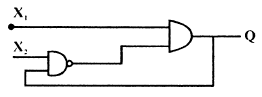Solution:

Let X1 = 1 & X2= 1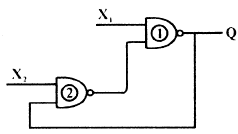IfQ=0 & X1=1

The o/P of gate -2, will be 1, and which will make Q = 1

if Q = 1 & X2 = 1, then 0/P of gate - 2 will be 0 and which will make output of gate - 1 Q = 0

*Answer can only contain numeric values
QUESTION: 9

The Minimum no. of 2 inputs NAND gate required to implement Boolean function f(A, B, C, D) =

ABCD are_______

Solution:

Correct Answer :- 3

Explanation :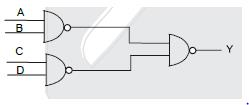QUESTION: 10

The 4 variable function f is given in terms of min term as f(A, B, C, D) = E(2,3,8,10,11,12,14,15)

Q. If by use of K-Map function is minimized in sum of product forms then SOP is

Solution: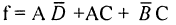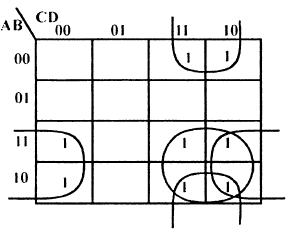QUESTION: 11

The 4 variable function f is given in terms of min term as f(A, B, C, D) = E(2,3,8,10,11,12,14,15)

Q. Above Minimised SOP, can be implemented by how many minm no. of 2 input NAND gate.

Solution:*Answer can only contain numeric values
QUESTION: 12

Consider the following data in respect of a certain digital gate

loH = 0.2 mA I1H= 40μ.A, 10L = 16 mA, liL = 1.6 mA symbols have their meaning. Fan out will be

Solution:

F.0 =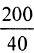= 5

Smalls of both will be Fan out

F.I =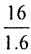=10

QUESTION: 13

If x, y and z are three Boolean variables, then F(x,y,z) = x + xy + y + yz + z + xz is equivalent to

Solution:
QUESTION: 14

How many AND gates are required for a 1-to-8 multiplexer?

Solution:

The number of AND gates required will be equal to the number of outputs in a demultiplexer.

QUESTION: 15

The logic circuit shown in figure is :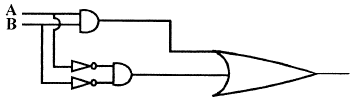Solution:
*Answer can only contain numeric values
QUESTION: 16

In a dual slope ADC if reference voltage is 100 mV and the first integration period is set as 50 msec.

For an input voltage of 120 mV, the second integration (de-integration) period is___ ms

Solution: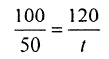t = 60 m sec

*Answer can only contain numeric values
QUESTION: 17

In a dual slope type digital voltmeter, an unknown signal voltage is integrated our 100 cycles of clock. If the signal has a 50 Hz pick up the maximum clock frequency can be__ kHz

Solution:

50 x 100

QUESTION: 18

In circuit given if both Transistors have same VT what is the approximate value of highest possible output voltage vout if vh, can range from 0 to VDD, it is assumed that 0 < vT < VDD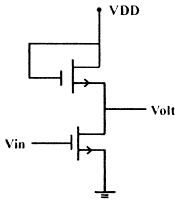Solution:
QUESTION: 19

In the I.C. logic gate shown in figure.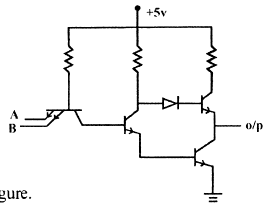If threshold voltage VBE is o.75 volt and VCE (sat) = 0.2 V, calculate. Value of output voltage

Q. If VA = VB = 4.5 volt

Solution:

It is A NAND gate and if VA= VB = 4.5
Then o/p will be logic zero, as both are logic high

SO V0 = VCE, sat = 0•2v

QUESTION: 20

If VA = 4.5 volt, VB = 0.2 volt

Solution:

if VA = 4.5v & VB = 0.2 v
so o/p will be 1, ie. Vo = 5 volt